# Elaborate on the output of learn_DAG() using get_ functions

library(BCDAG)

This is the third of a series of three vignettes for the R package BCDAG. In this vignette, we show how to use the output of learn_DAG() for posterior inference on DAGs, DAG parameters, and causal effect estimation. Specifically, we introduce the functions of the get_ family. Remember that the output of learn_DAG() consists of an MCMC sample from the marginal posterior distribution of DAG structures (collapse = TRUE) and the joint posterior of DAGs and DAG parameters (collapse = FALSE); see also the corresponding vignette

To start with, we simulate a dataset X from a randomly generated Gaussian DAG model as shown in an other vignette

## Generate data
set.seed(1)
q <- 8
w <- 0.2
DAG <- rDAG(q,w)
a <- q
U <- diag(1,q)
outDL <- rDAGWishart(n=1, DAG, a, U)
L <- outDL$L; D <- outDL$D
Omega <- L %*% solve(D) %*% t(L)
Sigma <- solve(Omega)
n <- 1000
X <- mvtnorm::rmvnorm(n = n, sigma = Sigma)

Next, we use learn_DAG() to approximate the joint posterior distribution over DAG structures and DAG parameters:

## Run MCMC
out <- learn_DAG(S = 5000, burn = 1000, data = X,
a, U, w,
fast = FALSE, save.memory = FALSE, collapse = FALSE)

## MCMC diagnsotics of convergence: function get_diagnostics()

Before using the MCMC output for posterior inference, it is common practice to perform some convergence checks. Function get_diagnostics() provides graphical diagnostics of convergence for the MCMC output of learn_DAG(). These are based on: the number of edges in the DAGs; the posterior probability of edge inclusion for each possible edge $$u \rightarrow v$$, both monitored across MCMC iterations. Input of the function is an object of class bcdag and the output consists of:

• a traceplot and running-mean plot of the number of edges in the DAGs (graph size);

• a collection of traceplots of the posterior probabilities of edge inclusion computed across MCMC iterations.

For each pair of distinct nodes $$(u,v)$$, its posterior probability of inclusion at time $$s$$ $$(s = 1,\dots, S)$$ is estimated as the proportion of DAGs visited by the MCMC up to time $$s$$ which contain the directed edge $$u \rightarrow v$$. Output is organized in $$q$$ plots (one for each node $$v = 1, \dots, q$$), each summarizing the posterior probabilities of edges $$u \rightarrow v$$, $$u = 1,\dots, q$$.

get_diagnostics(out)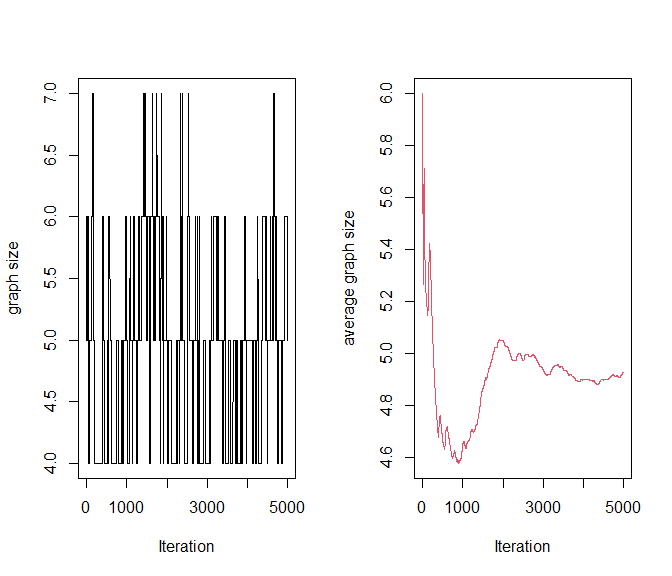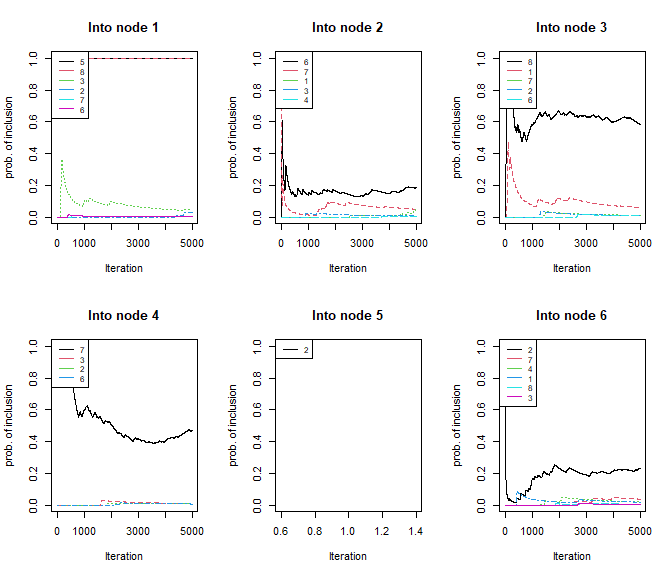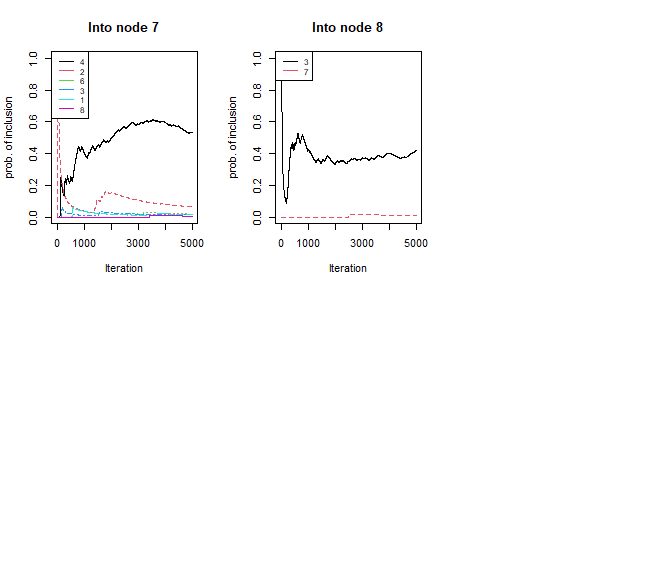## Posterior inference: DAG structure learning

We now show how to perform posterior inference of DAGs from the MCMC output. To summarize the output of learn_DAG(), a summary() method for objects of class bcdag is available. When summary() is applied to a bcdag object, a printed message appears. This summarizes the type of bcdag object (see details on bcdag object types provided in the previous vignette) and the input arguments of learn_DAG() that generated the output. In addition, the function returns some graphical outputs representing the Median Probability DAG Model estimate (MPM), the estimated posterior probabilities of edge inclusion and the posterior distribution of the graph size:

summary(out)
#> A  complete  bcdag object containing  5000  draws from the joint posterior over DAGs, L and D. (Burnin = 1000 ).
#>
#> Prior hyperparameters:
#> w =  0.2
#> a =  8
#> U =     [,1] [,2] [,3] [,4] [,5] [,6] [,7] [,8]
#> [1,]    1    0    0    0    0    0    0    0
#> [2,]    0    1    0    0    0    0    0    0
#> [3,]    0    0    1    0    0    0    0    0
#> [4,]    0    0    0    1    0    0    0    0
#> [5,]    0    0    0    0    1    0    0    0
#> [6,]    0    0    0    0    0    1    0    0
#> [7,]    0    0    0    0    0    0    1    0
#> [8,]    0    0    0    0    0    0    0    1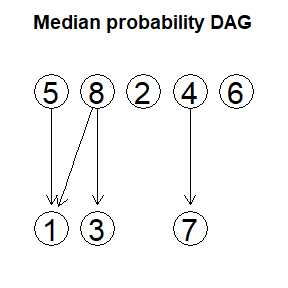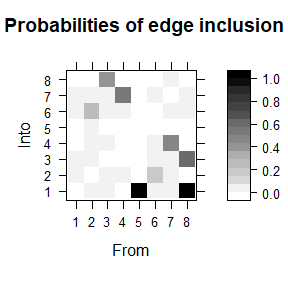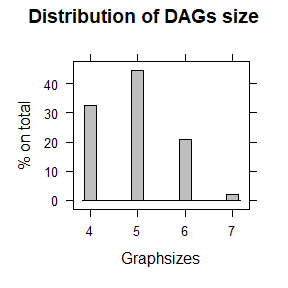Function get_edgeprobs() computes and returns the collection of posterior probabilities of edge inclusion, arranged as a $$(q,q)$$ matrix, with $$(u,v)$$-element referring to edge $$u\rightarrow v$$:

get_edgeprobs(out)
#>        1      2      3      4      5      6      7      8
#> 1 0.0000 0.0480 0.0596 0.0000 0.0000 0.0182 0.0158 0.0000
#> 2 0.0262 0.0000 0.0092 0.0064 0.0018 0.2270 0.0638 0.0000
#> 3 0.0474 0.0064 0.0000 0.0096 0.0000 0.0042 0.0162 0.4162
#> 4 0.0000 0.0040 0.0000 0.0000 0.0000 0.0204 0.5328 0.0000
#> 5 1.0000 0.0000 0.0000 0.0000 0.0000 0.0000 0.0000 0.0000
#> 6 0.0020 0.1864 0.0084 0.0064 0.0000 0.0000 0.0186 0.0000
#> 7 0.0044 0.0494 0.0106 0.4672 0.0000 0.0372 0.0000 0.0092
#> 8 1.0000 0.0000 0.5838 0.0000 0.0000 0.0054 0.0060 0.0000

The MPM model returned in the output of summary() can be used as a single DAG-model estimate and is obtained by including all edges whose posterior probability exceeds the threshold $$0.5$$. Function get_MPMdag() applies to an object of class bcdag and returns the $$(q,q)$$ adjacency matrix of the MPM:

MPMdag <- get_MPMdag(out)
MPMdag
#>   1 2 3 4 5 6 7 8
#> 1 0 0 0 0 0 0 0 0
#> 2 0 0 0 0 0 0 0 0
#> 3 0 0 0 0 0 0 0 0
#> 4 0 0 0 0 0 0 1 0
#> 5 1 0 0 0 0 0 0 0
#> 6 0 0 0 0 0 0 0 0
#> 7 0 0 0 0 0 0 0 0
#> 8 1 0 1 0 0 0 0 0

As an alternative, the Maximum A Posterior DAG estimate (MAP) can be considered. This corresponds to the DAG with the highest MCMC frequency of visits and can be recovered through the function get_MAPdag():

MAPdag <- get_MAPdag(out)
MAPdag
#>   1 2 3 4 5 6 7 8
#> 1 0 0 0 0 0 0 0 0
#> 2 0 0 0 0 0 0 0 0
#> 3 0 0 0 0 0 0 0 0
#> 4 0 0 0 0 0 0 0 0
#> 5 1 0 0 0 0 0 0 0
#> 6 0 0 0 0 0 0 0 0
#> 7 0 0 0 1 0 0 0 0
#> 8 1 0 1 0 0 0 0 0
par(mfrow = c(1,3))
gRbase::plot(as(DAG, "graphNEL"), main = "True DAG")
gRbase::plot(as(MPMdag, "graphNEL"), main = "MPM DAG")
gRbase::plot(as(MAPdag, "graphNEL"), main = "MAP DAG")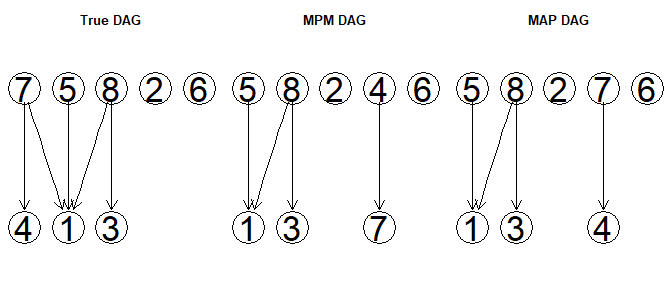In this example, the MPM and MAP estimates differ by a single an edge between nodes $$4$$ and $$7$$ which is reversed among the two graphs. However, it can be shown that the two DAG estimates are Markov equivalent, meaning that they encode the same conditional independencies between variables. In a Gaussian setting, Markov equivalent DAGs cannot be distinguished with observational data as they represent the same statistical model. Therefore, there is no difference in choosing the MPM or the MAP estimate to infer the structure of dependencies between variables.

In addition, if compared with the true graph, the DAG estimate provided by MPM [CORRETTO?] differs by a single edge between nodes $$7$$ and $$1$$ which is missing from MPM. Interestingly, one can see that the regression coefficient associated with $$u\rightarrow v$$, $$\boldsymbol L_{7,1}$$, is relatively “small”, implying that the strength of the dependence between the two nodes is “weak”:

round(L, 3)
#>        [,1] [,2]  [,3]  [,4] [,5] [,6] [,7] [,8]
#> [1,]  1.000    0 0.000  0.00    0    0    0    0
#> [2,]  0.000    1 0.000  0.00    0    0    0    0
#> [3,]  0.000    0 1.000  0.00    0    0    0    0
#> [4,]  0.000    0 0.000  1.00    0    0    0    0
#> [5,] -0.018    0 0.000  0.00    1    0    0    0
#> [6,]  0.000    0 0.000  0.00    0    1    0    0
#> [7,] -0.006    0 0.000 -1.89    0    0    1    0
#> [8,]  0.373    0 0.474  0.00    0    0    0    1

## Posterior inference: causal effect estimation

In this last section, we introduce functions causaleffect() and get_causaleffect(), which allow to compute and estimate causal effects between variables. Specifically, we consider the causal effect on a response variable of interest consequent to a joint intervention on a given set of variables; see also Nandy et al. (2017) and Castelletti & Mascaro (2021) for formal definitions.

For a given DAG, it is possible to identify and estimate the causal effect on a node $$Y$$ consequent to a hypothetical hard intervention on node $$j$$ using the rules of the do-calculus (Pearl, 2000). A simple implementation of this set of rules and an estimation method for the causally sufficient case and for Gaussian data is provided by function causaleffect(). The function takes as input a numerical vector representing the labels of the intervened nodes (also called intervention target), a numerical value indicating the response variable and the DAG model parameters L and D; see also Castelletti & Mascaro (2021) or our previous vignette for a detailed model description.

For a given response variable $$Y \in\{1, \ldots, q\}$$, and intervention target $$I \subseteq \{1,\dots,q\}$$ the total joint effect of an intervention $$\operatorname{do}\left\{X_{j}=\tilde{x}_{j}\right\}_{j \in I}$$ on $$Y$$ is $\theta_{Y}^{I}:=\left(\theta_{h, Y}^{I}\right)_{h \in I},$ where for each $$h \in I$$ $\theta_{h, Y}^{I}:=\frac{\partial}{\partial x_{h}} \mathbb{E}\left(Y \mid \operatorname{do}\left\{X_{j}=\tilde{x}_{j}\right\}_{j \in I}\right)$

See also Castelletti & Mascaro (2021) and Nandy et al. (2017) for more details.

To better understand the difference between single and joint interventions, consider as an example the total causal effect on node $$Y=1$$ (i.e. variable $$X_1$$) of a joint intervention on nodes $$4$$ and $$5$$, $$I=\{4,5\}$$ (i.e. variables $$X_4, X_5$$) and the total causal effects of two separate interventions on nodes $$4$$ and $$5$$ under the causal model represented by the DAG generated before:

gRbase::plot(as(DAG, "graphNEL"), main = "True DAG")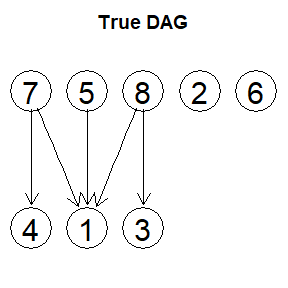These are given by:

causaleffect(targets = c(4,5), response = 1, L = L, D = D)
#>  0.00000000 0.01777791
causaleffect(targets = 4, response = 1, L = L, D = D)
#>  0
causaleffect(targets = 5, response = 1, L = L, D = D)
#>  0.01777791

As it can be observed, the total causal effect of intervening on variable $$X_4$$ is null both in a single intervention on $$4$$ and in a joint intervention on $$\{X_4, X_5\}$$, while intervening on $$X_5$$ produces the same positive total causal effect in both cases. The total causal effects produced are thus exactly the same for both variables in the two cases. However, if we slightly modify the DAG by adding an edge from node $$4$$ to node $$5$$, so that:

DAG2 <- DAG
DAG2[4,5] <- 1
par(mfrow = c(1,2))
gRbase::plot(as(DAG, "graphNEL"), main = "True DAG")
gRbase::plot(as(DAG2, "graphNEL"), main = "Modified DAG")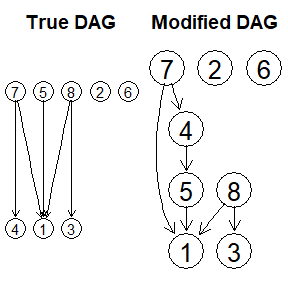and modify L accordingly:

L2 <- L
L2[4,5] <- runif(1)
L2[4,5]
#>  0.5357392

The comparison of single and joint total causal effects now produces different results:

causaleffect(targets = c(4,5), response = 1, L = L2, D = D)
#>  0.00000000 0.01777791
causaleffect(targets = 4, response = 1, L = L2, D = D)
#>  -0.009524322
causaleffect(targets = 5, response = 1, L = L2, D = D)
#>  0.01777791

As it can be observed, this time a single intervention on $$X_4$$ produces a negative causal effect on $$X_1$$, while jointly intervening on $$X_4$$ and $$X_5$$ makes the total causal effect of $$X_4$$ on $$X_1$$ null. The effect of $$X_4$$ on $$X_1$$ was in fact mediated by $$X_5$$: intervening simultaneously also on $$X_5$$ erases the effect of $$X_4$$ on $$X_5$$ and, in turn, of $$X_4$$ on $$X_1$$. See also Castelletti & Mascaro (2021) or Nandy et al. (2017) for a more detailed description.

The identification and estimation of causal effects requires the specification of a DAG. When the DAG is unknown, function get_causaleffect() can be used. It applies to objects of class bcdag; the latter corresponds to the output of learn_DAG() and consists of a sample of size $$S$$ from the posterior of DAGs and DAG parameters. In addition get_causaleffect() takes as input a numerical vector representing the labels of the intervened nodes (the intervention target) and a numerical value indicating the response variable. Output of the function is a sample of size $$S$$ from the posterior distribution of the causal effect coefficients associated with the intervention targets:

effects_out <- get_causaleffect(out, targets = c(4,5), response = 1)
#>      h = 4      h = 5
#> [1,]     0 0.01940036
#> [2,]     0 0.01435828
#> [3,]     0 0.01852334
#> [4,]     0 0.01807825
#> [5,]     0 0.01956232
#> [6,]     0 0.01922416

Additionally, if BMA = TRUE, get_causaleffect() returns a Bayesian Model Average (BMA) estimate of the causal effect coefficients:

## BMA estimate of causal effects
round(get_causaleffect(out, targets = c(4,5), response = 1, BMA = TRUE), 3)
#> h = 4 h = 5
#> 0.000 0.018
## True causal effects
round(causaleffect(targets = c(4,5), response = 1, L = L, D = D), 3)
#>  0.000 0.018

Also notice that, if the BCDAG object input of get_causaleffect() is of type collapsed or compressed and collapsed, then get_causaleffect() requires drawing from the posterior distribution of parameters $$(\boldsymbol L, \boldsymbol D)$$ before estimating the required causal effects:

coll_out <- learn_DAG(S = 5000, burn = 1000, data = X,
a, U, w,
fast = FALSE, save.memory = FALSE, collapse = TRUE)
names(coll_out)
#>  "Graphs"
effects_collout <- get_causaleffect(coll_out, targets = c(4,5), response = 1, BMA = TRUE)
round(effects_collout, 3)
#> h = 4 h = 5
#> 0.000 0.018